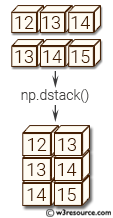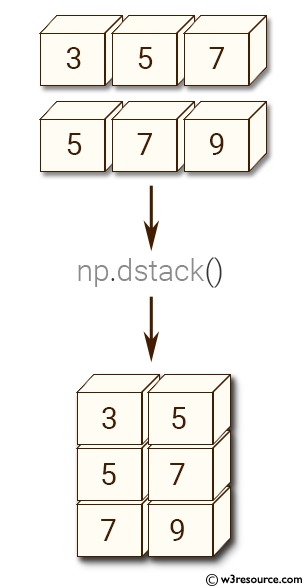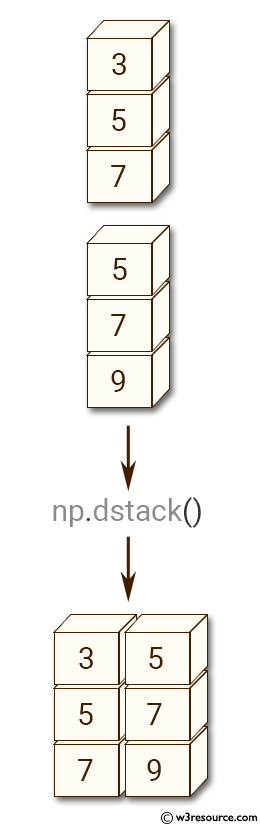# NumPy Array manipulation: dstack() function

## numpy.dstack() function

The dstack() is used to stack arrays in sequence depth wise (along third axis).
This is equivalent to concatenation along the third axis after 2-D arrays of shape (M,N) have been reshaped to (M,N,1) and 1-D arrays of shape (N,) have been reshaped to (1,N,1). Rebuilds arrays divided by dsplit.
This function makes most sense for arrays with up to 3 dimensions. For instance, for pixel-data with a height (first axis), width (second axis), and r/g/b channels (third axis). The functions concatenate, stack and block provide more general stacking and concatenation operations.

Syntax:

`numpy.dstack(tup)`Version: 1.15.0

Parameter:

Name Description Required /
Optional
tup The arrays must have the same shape along all but the third axis. 1-D or 2-D arrays must have the same shape. Required

Return value:

stacked : ndarray The array formed by stacking the given arrays, will be at least 3-D.

Example-1: numpy.dstack()

``````>>> import numpy as np
>>> x = np.array((3, 5, 7))
>>> y = np.array((5, 7, 9))
>>> np.dstack((x,y))
array([[[3, 5],
[5, 7],
[7, 9]]])
``````

Pictorial Presentation:Example-2: numpy.dstack()

``````>>> import numpy as np
>>> x = np.array([, , ])
>>> y = np.array([, , ])
>>> np.dstack((x,y))
array([[[3, 5]],

[[5, 7]],

[[7, 9]]])
``````

Pictorial Presentation:Python - NumPy Code Editor:

Previous: column_stack()
Next: hstack()

﻿